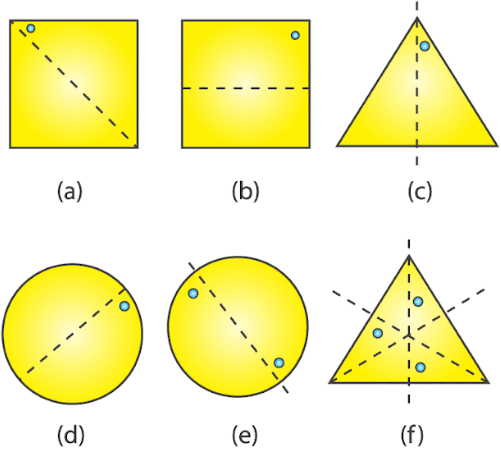# RD Sharma Solutions For Class 7 Maths Exercise 18.2 Chapter 18 Symmetry

In order to succeed in the exams, our expert team has provided the solutions to RD Sharma Class 7 Maths Chapter 18 in a well-structured manner with appropriate explanations for a better understanding of the concepts. Learners can download the pdf of RD Sharma Solutions for class 7 from the links provided below. Experts suggest students practice the solutions many numbers of times to yield good results in their exams. This exercise deals with lines of symmetry and reflection.

## Download the PDF of RD Sharma Solutions For Class 7 Chapter 18 – Symmetry Exercise 18.2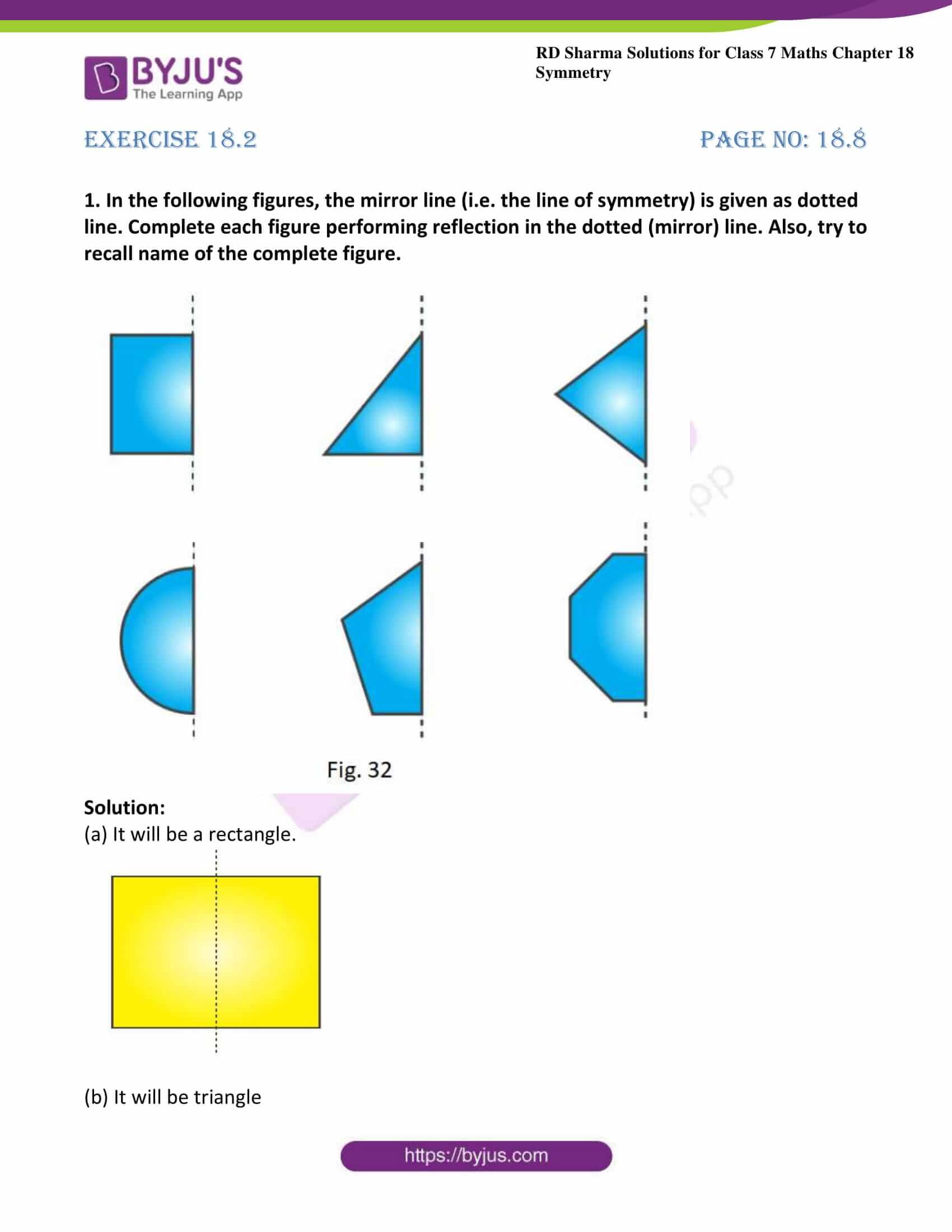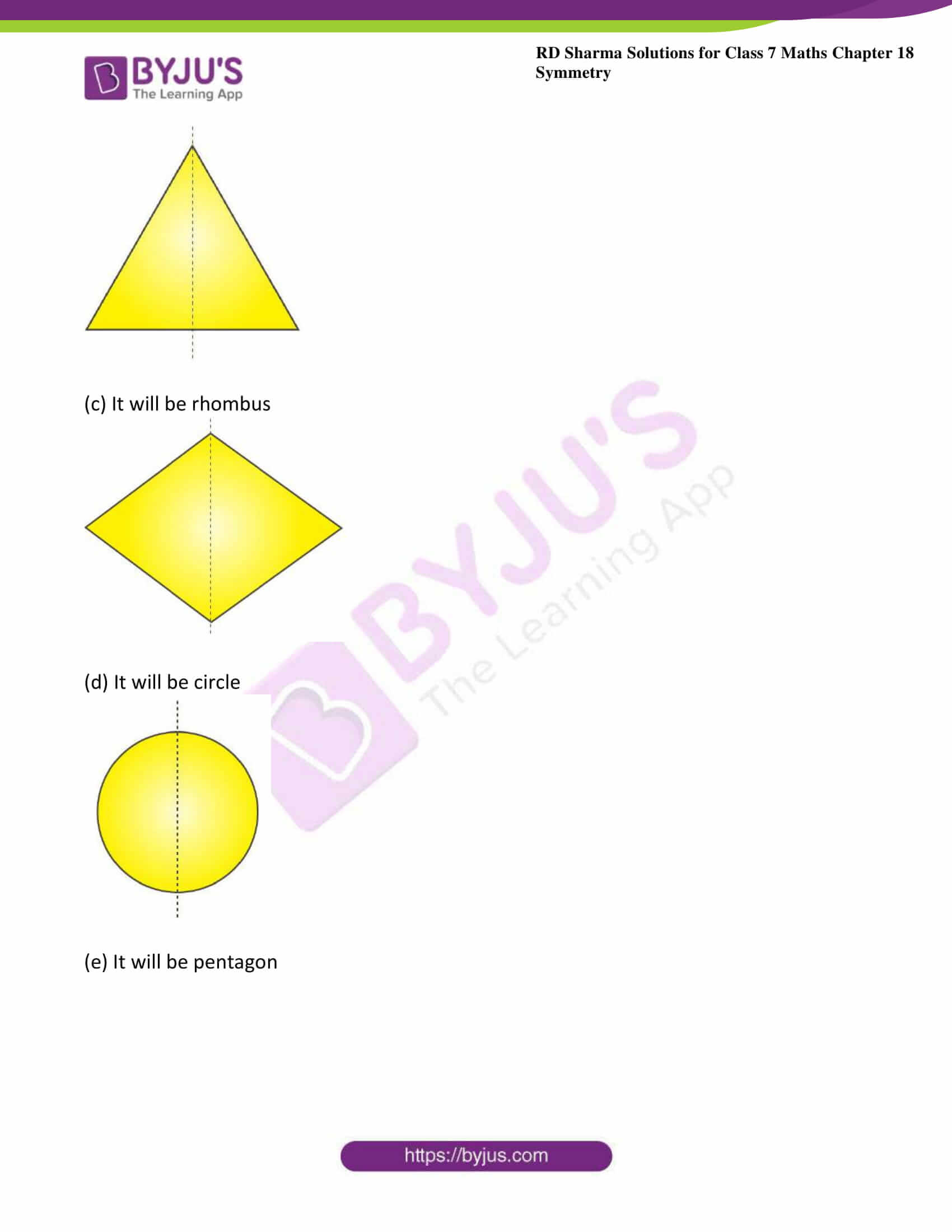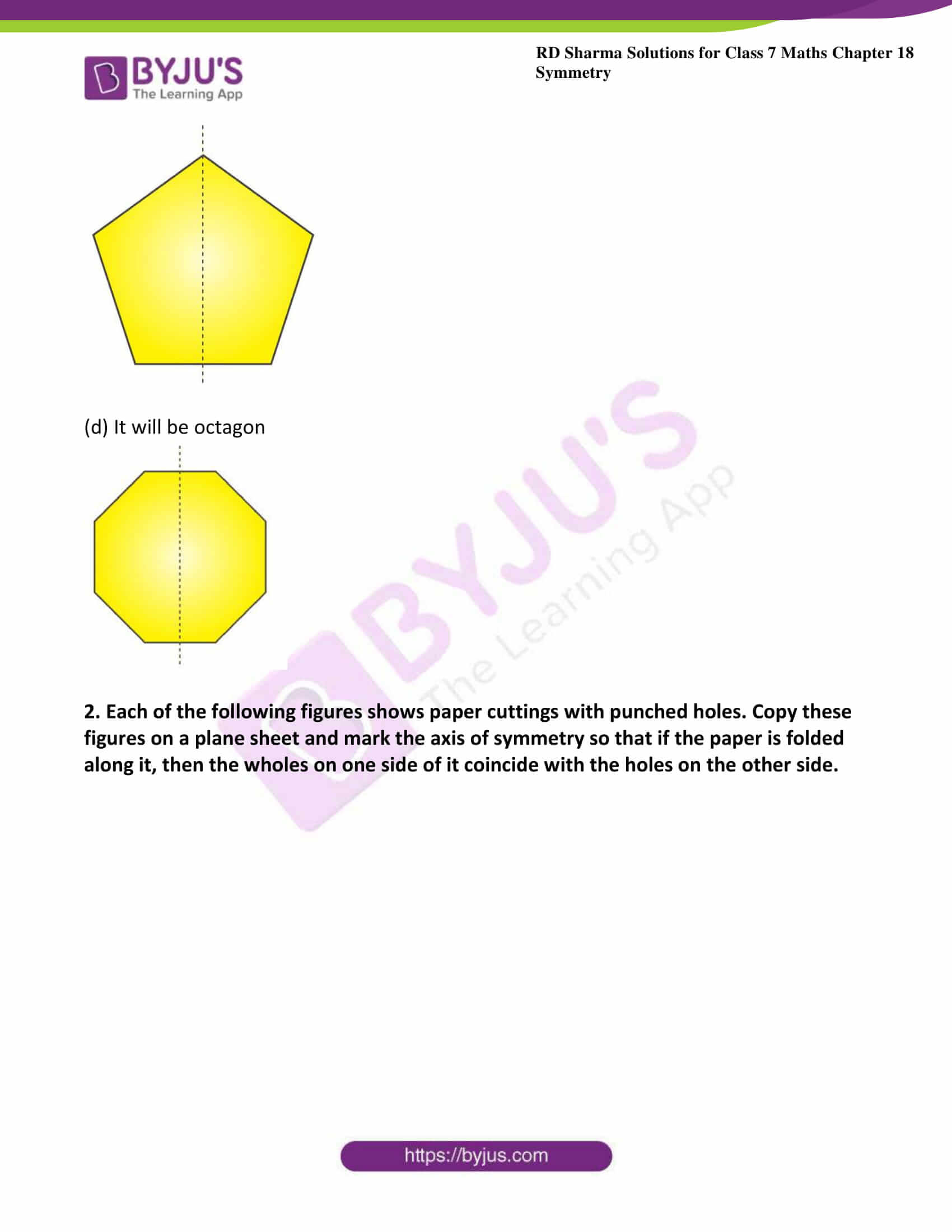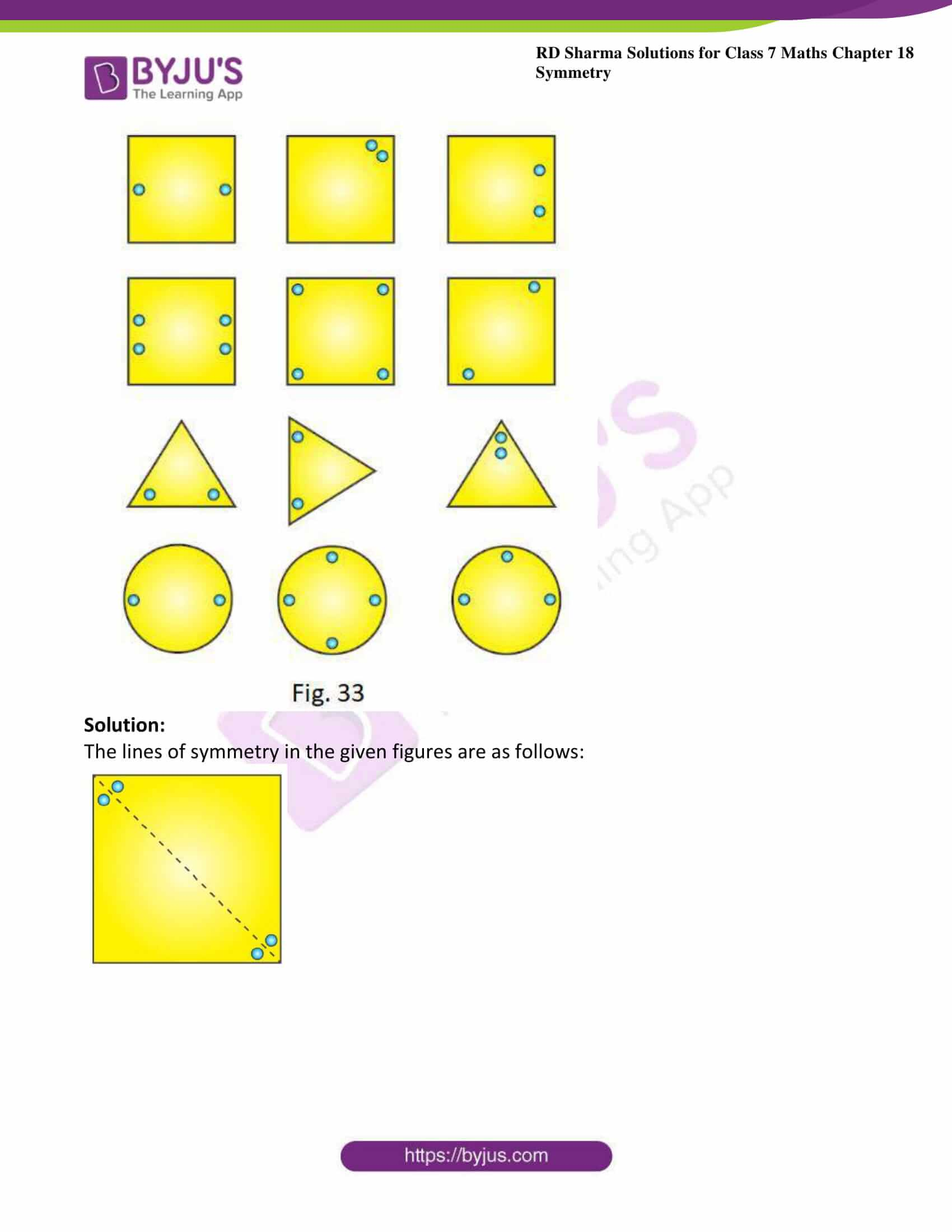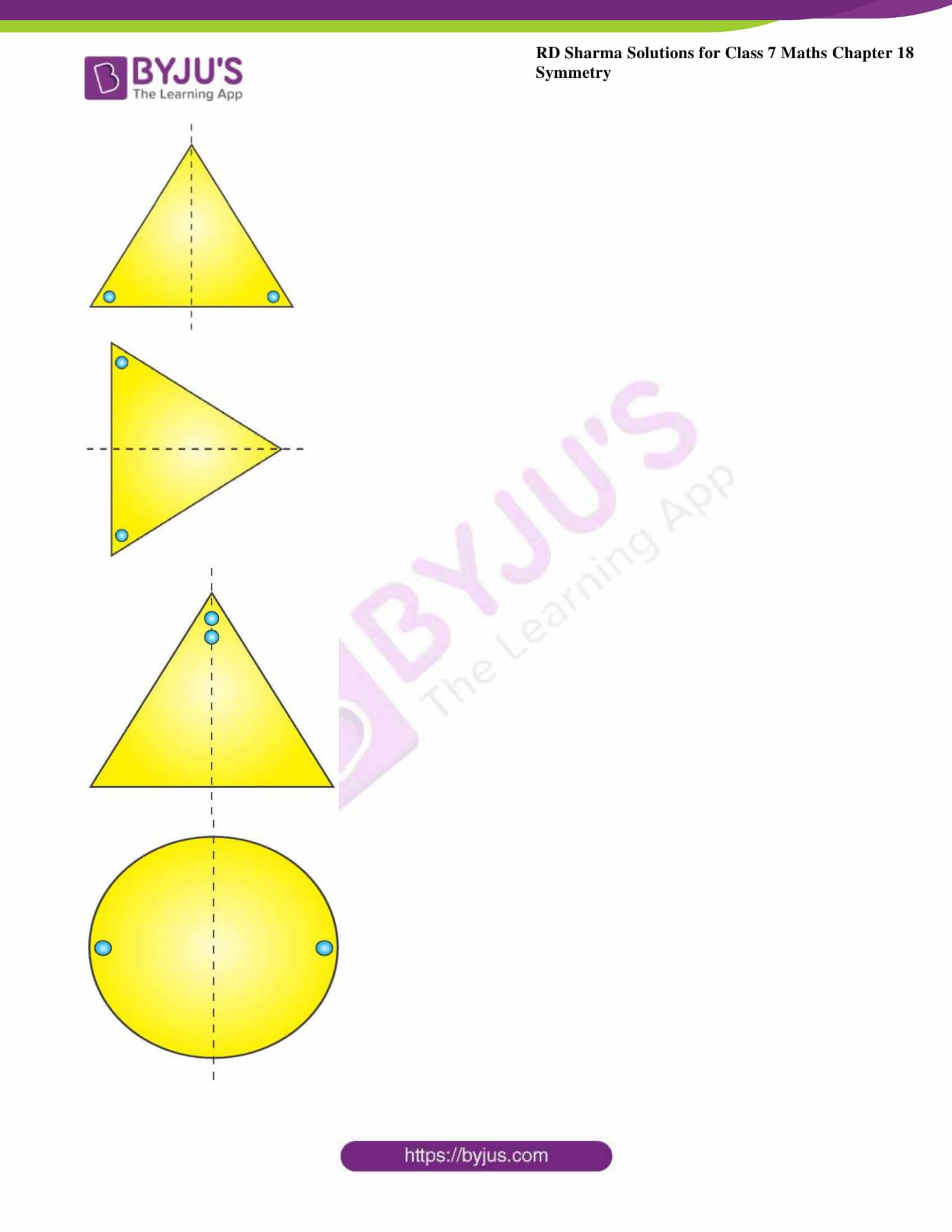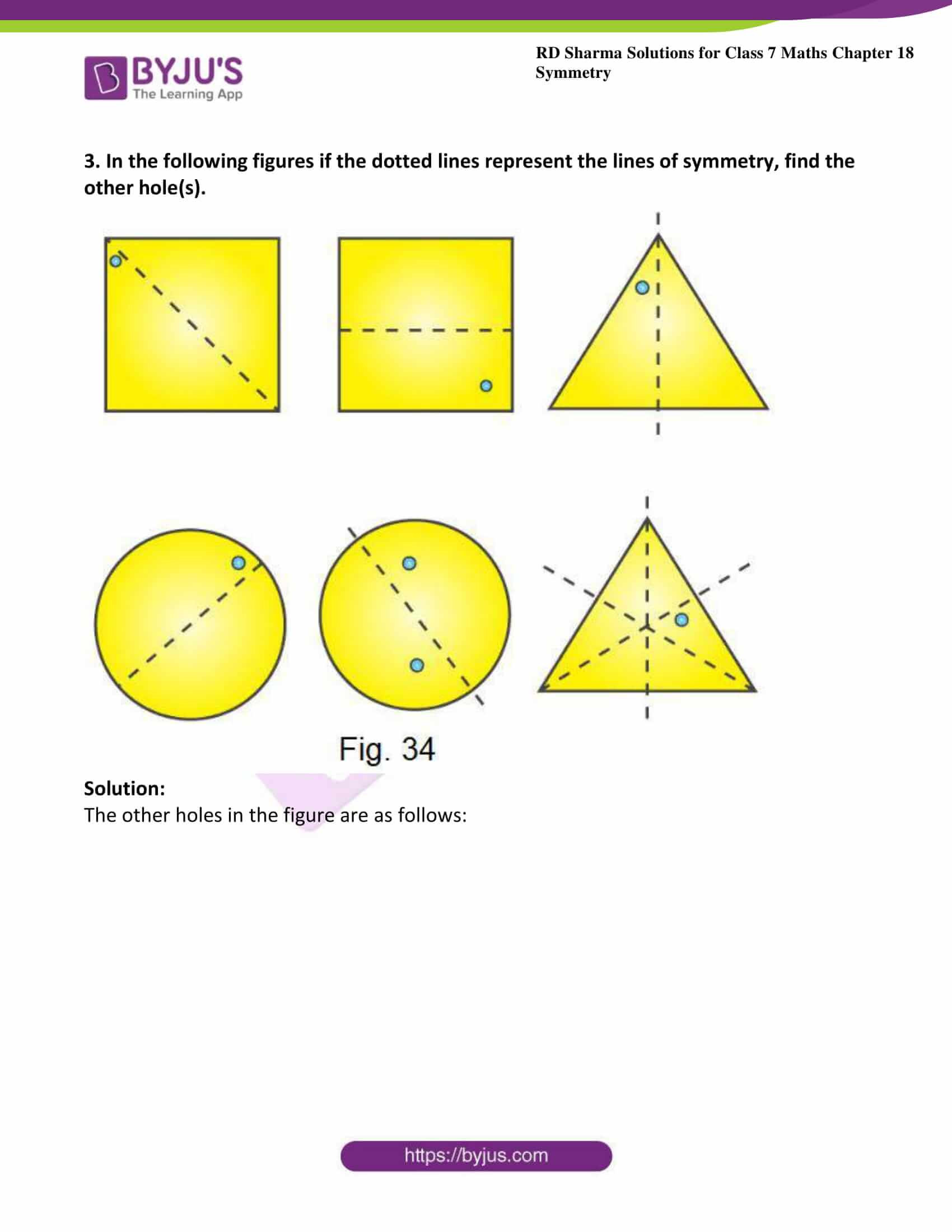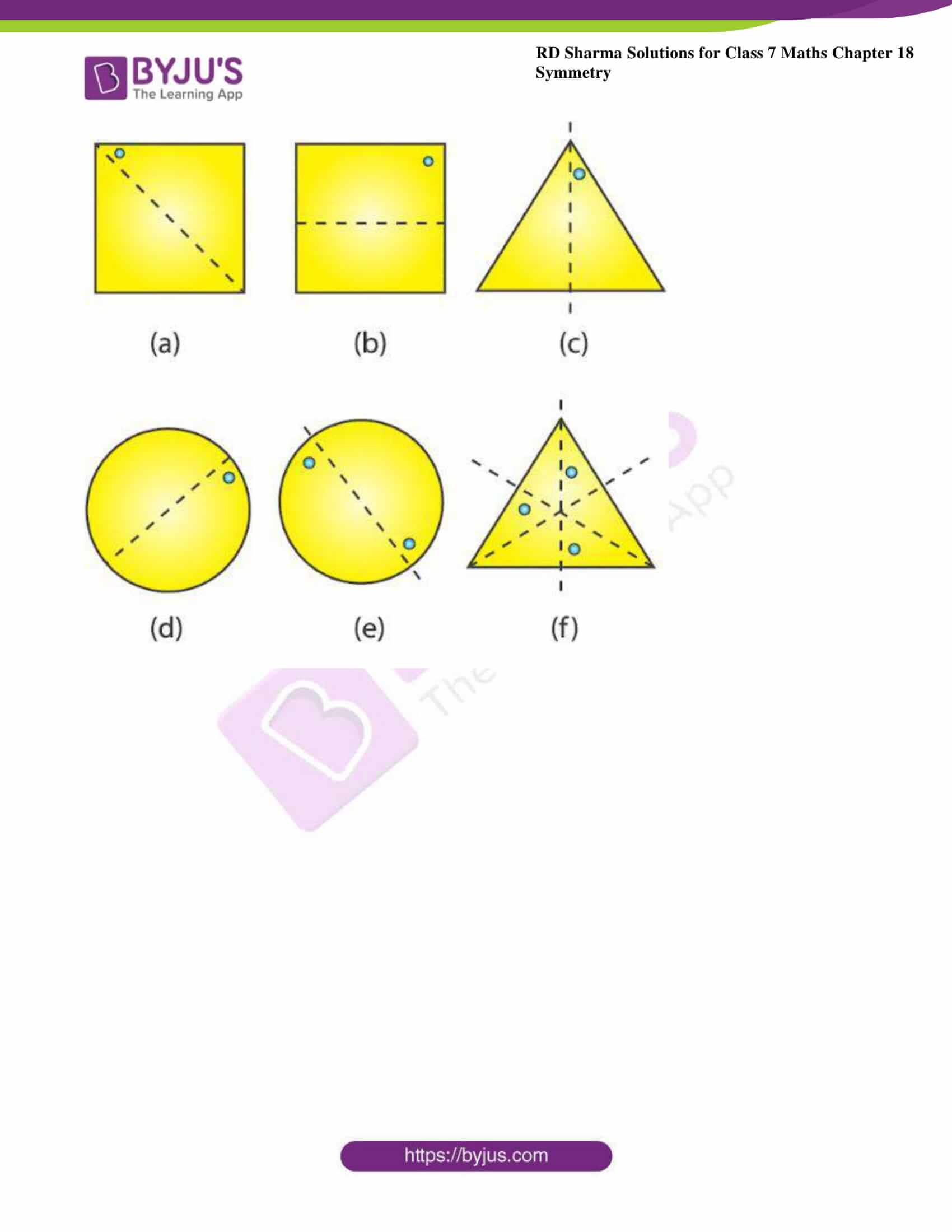### Access answers to Maths RD Sharma Solutions For Class 7 Chapter 18 – Symmetry Exercise 18.2

1. In the following figures, the mirror line (i.e. the line of symmetry) is given as dotted line. Complete each figure performing reflection in the dotted (mirror) line. Also, try to recall name of the complete figure.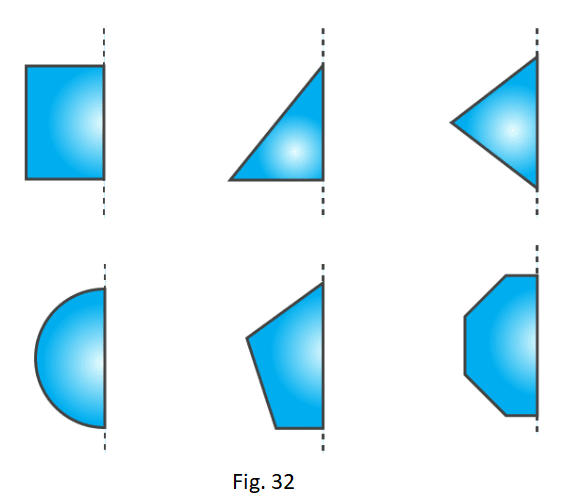Solution:

(a) It will be a rectangle.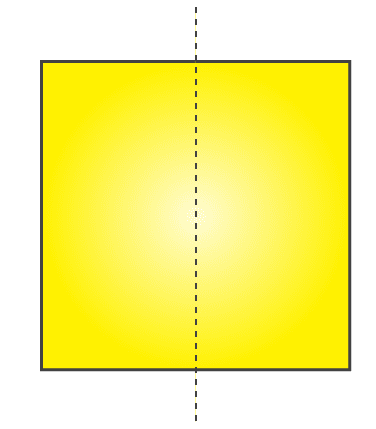(b) It will be triangle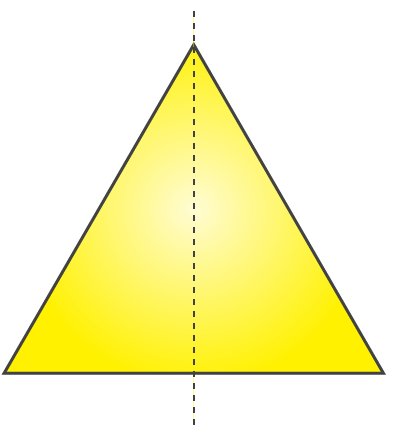(c) It will be rhombus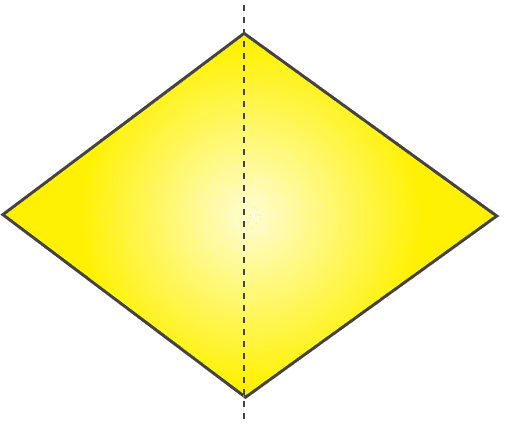(d) It will be circle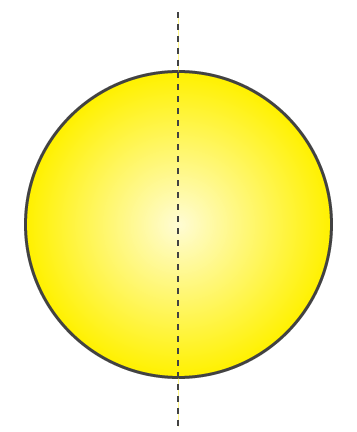(e) It will be pentagon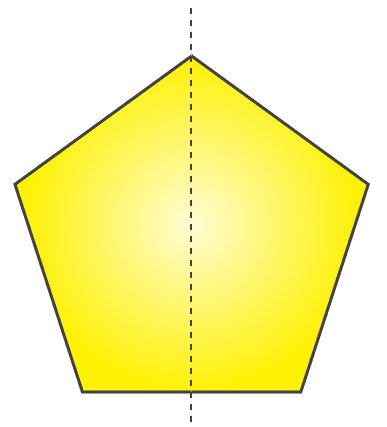(d) It will be octagon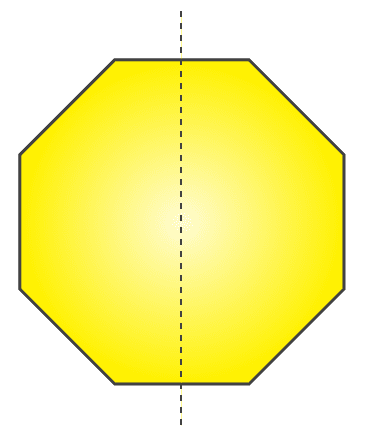2. Each of the following figures shows paper cuttings with punched holes. Copy these figures on a plane sheet and mark the axis of symmetry so that if the paper is folded along it, then the wholes on one side of it coincide with the holes on the other side.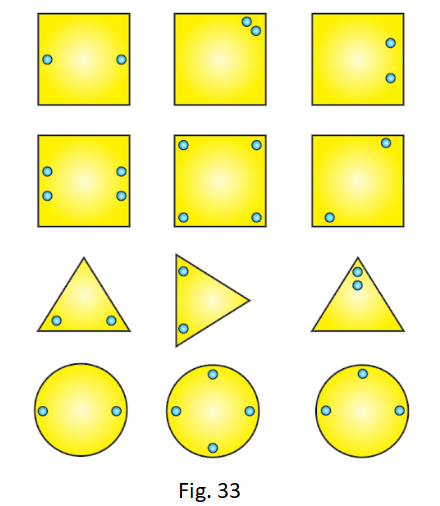Solution:

The lines of symmetry in the given figures are as follows: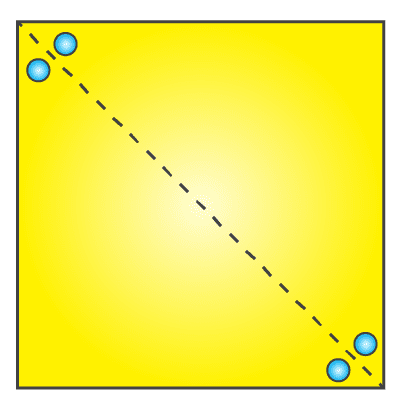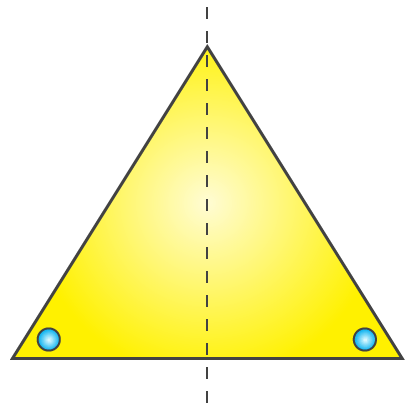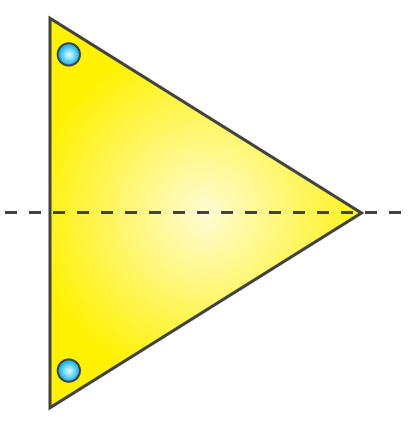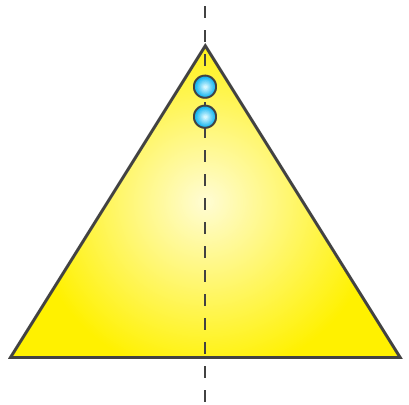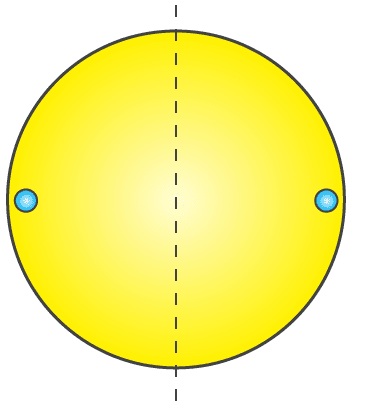3. In the following figures if the dotted lines represent the lines of symmetry, find the other hole(s).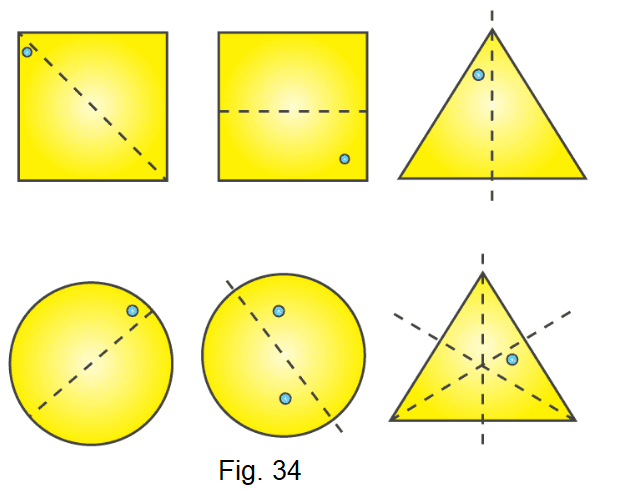Solution:

The other holes in the figure are as follows: